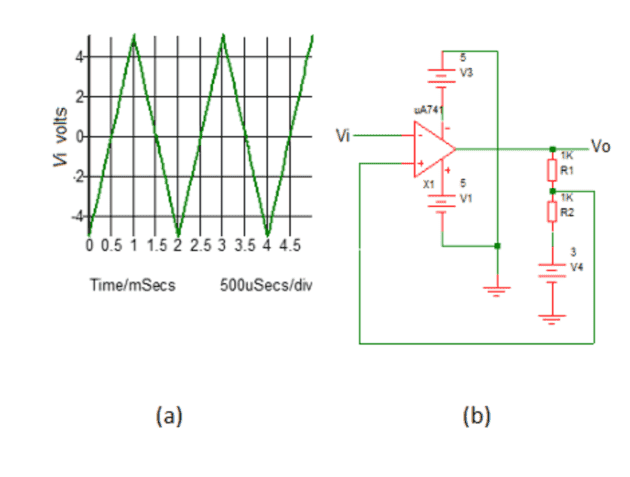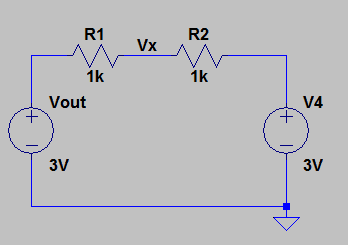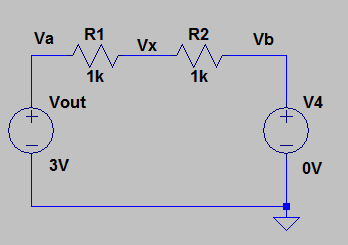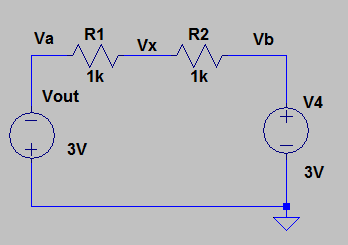# Help with voltage threshold calculations

Thread moved from the technical forums, so no HH Template is shown.
Please can someone tell me if this calculation is correct? Please see attached question.
I have used the +/-3V as the saturation voltage and multiplied it by R2/R2+R3 to give me +/-1.5V.

#### Attachments

• Threshold voltage question.docx
43 KB · Views: 314

Is this a Homework ?Notice that you have additional +3V voltage source (V4) in your circuit. And this voltage source will have big influence on threshold voltage.

Sorry yes, I think I may have posted it in the wrong place. First time using this site. I will have a rethink and post it back in the correct section.
Thank you very much for your help.

So now, can you show us the "right" answer? And my tip is: Find the voltage at non inverting input for two cases: one for Vout = +3V and Vout = -3V. And do not forget about V4.

Struggling to find the formula I should be using. I think this is a Schmitt Trigger and all calculations I have seen include a third feedback resistor which isn't here.

OK. But are you able to find the Vx voltage for this circuit?Would it be:
Vx = R1/R1+R2 x Vout = 0.5 x 3 = 1.5V?

No, wrong again? Why do you keep ignoring the V4 source?

OK using basic ohms law:
I = 3/1000 = 3mA
So voltage dropped across the 1K resistor would be 9mV making Vx =2.991 V?

OK, let us start again from the beginning.
The circuit look like this:And notice that now V4 = 0V and R1 and R2 are connected in series.
The voltage at Va is equal to Vout = 3V, the Vb voltage is 0V because V4 = 0V.
So the voltage across (R1+R2) is Va - Vb = 3V - 0V = 3V and the current is I = 3V/(R1+R2) = 3V/2kΩ = 1.5mA therefore Vx = Vout - I*R1 = 3V - 1.5V = 1.5V
But in your circuit V4 is not 0V it is 3V so the situation looks like this (for Vout = +3V)And when Vout = -3VCan you solve it for Vx ?

And I highly recommend you to read this by starting at page 3
http://www.ittc.ku.edu/~jstiles/312/handouts/312_Introduction_package.pdf

#### Attachments

•BigMan52
OK thank you very much.
So here goes:
Vx when Vout is -3V:
Vb =V4 = 3V
Voltage across R1+R2 = Va-Vb = -3-3 = -6V
Current I = -6/2000 = -3mA
Vx = Vout - (I x R1) = -3 - -3 = 0V

Does that mean the upper threshold voltage for this op amp is +3V and the lower threshold voltage is 0V?

Does that mean the upper threshold voltage for this op amp is +3V and the lower threshold voltage is 0V?
Yes exactly, this additional source (V4) will "shift" the threshold voltage up or down depend on V4 sign.

•BigMan52
Brilliant, thank you very much for all your help!